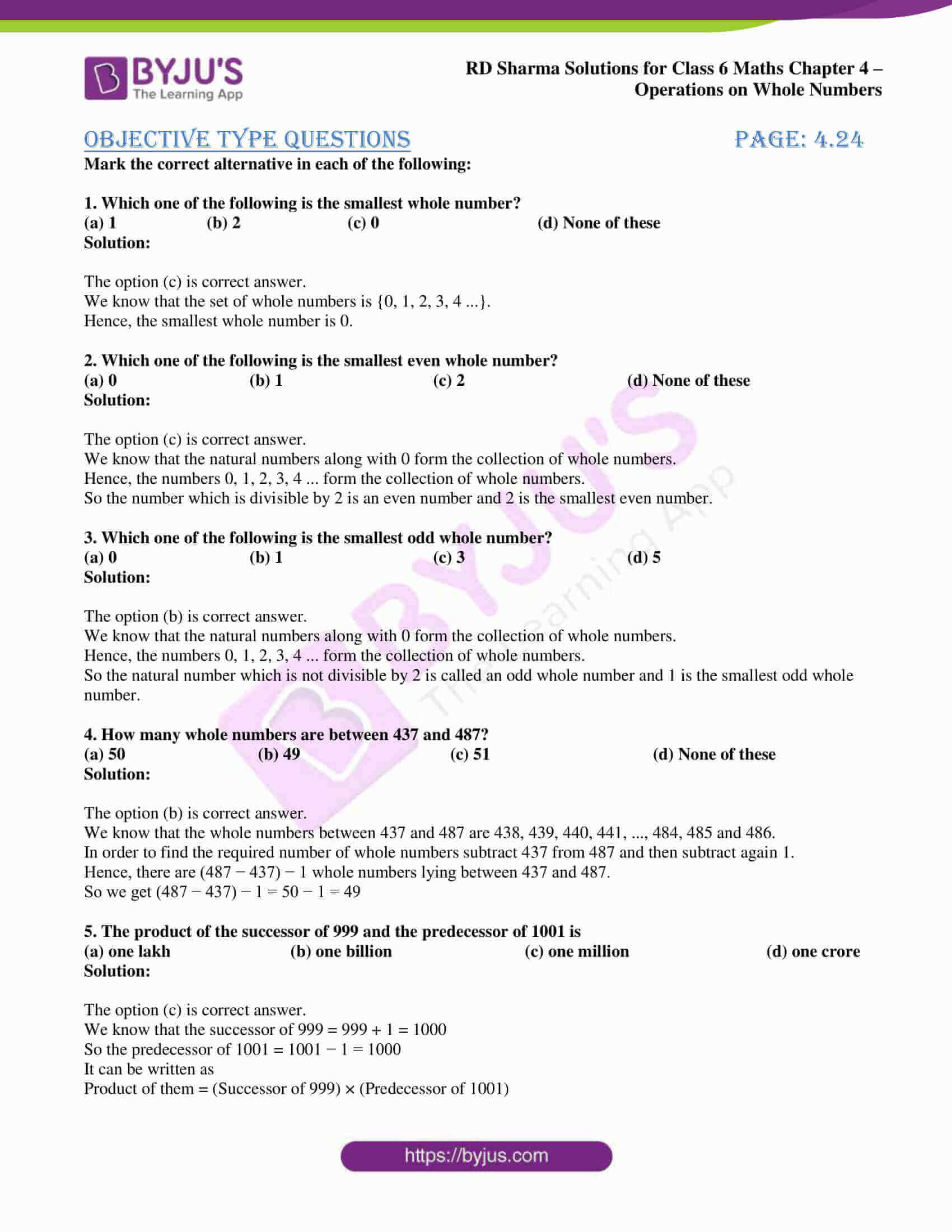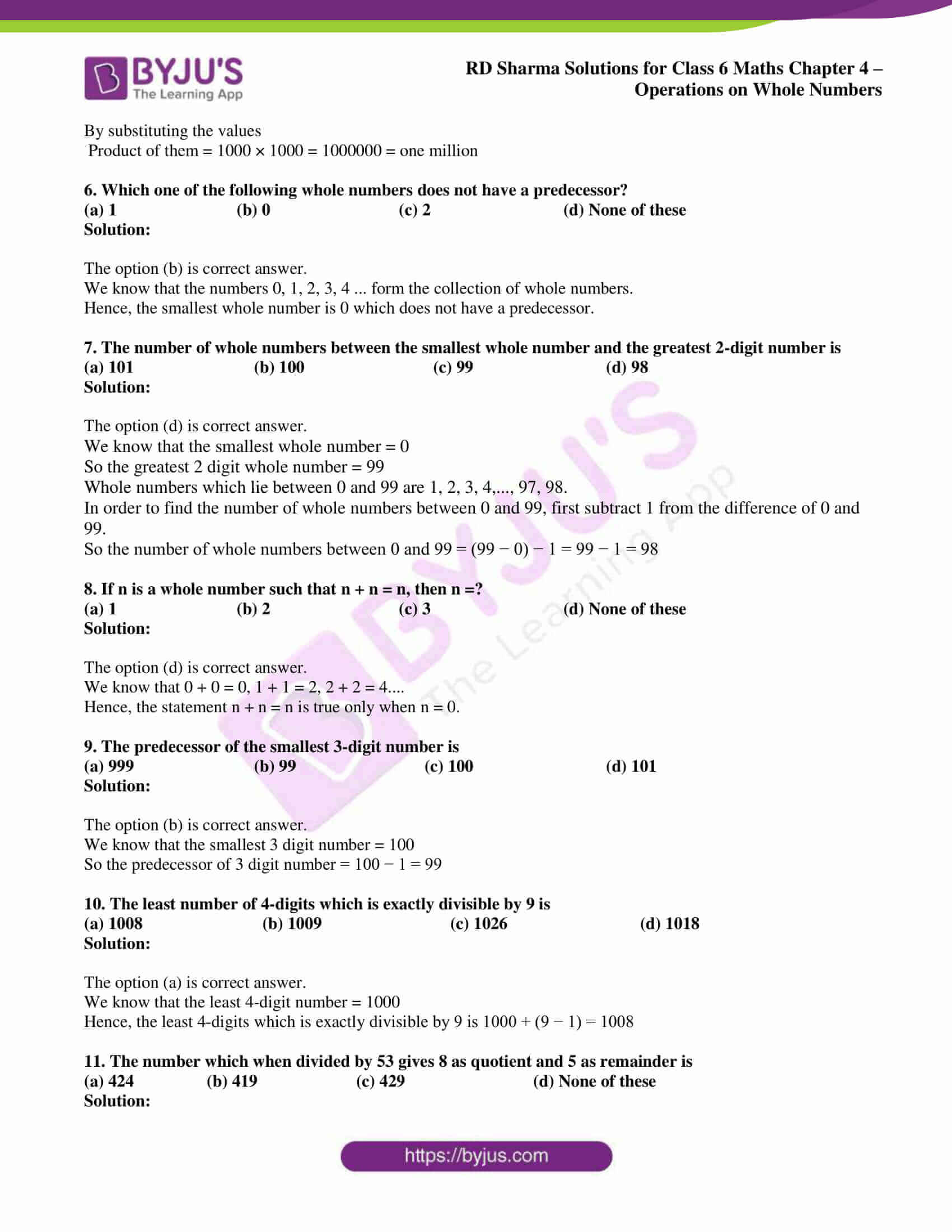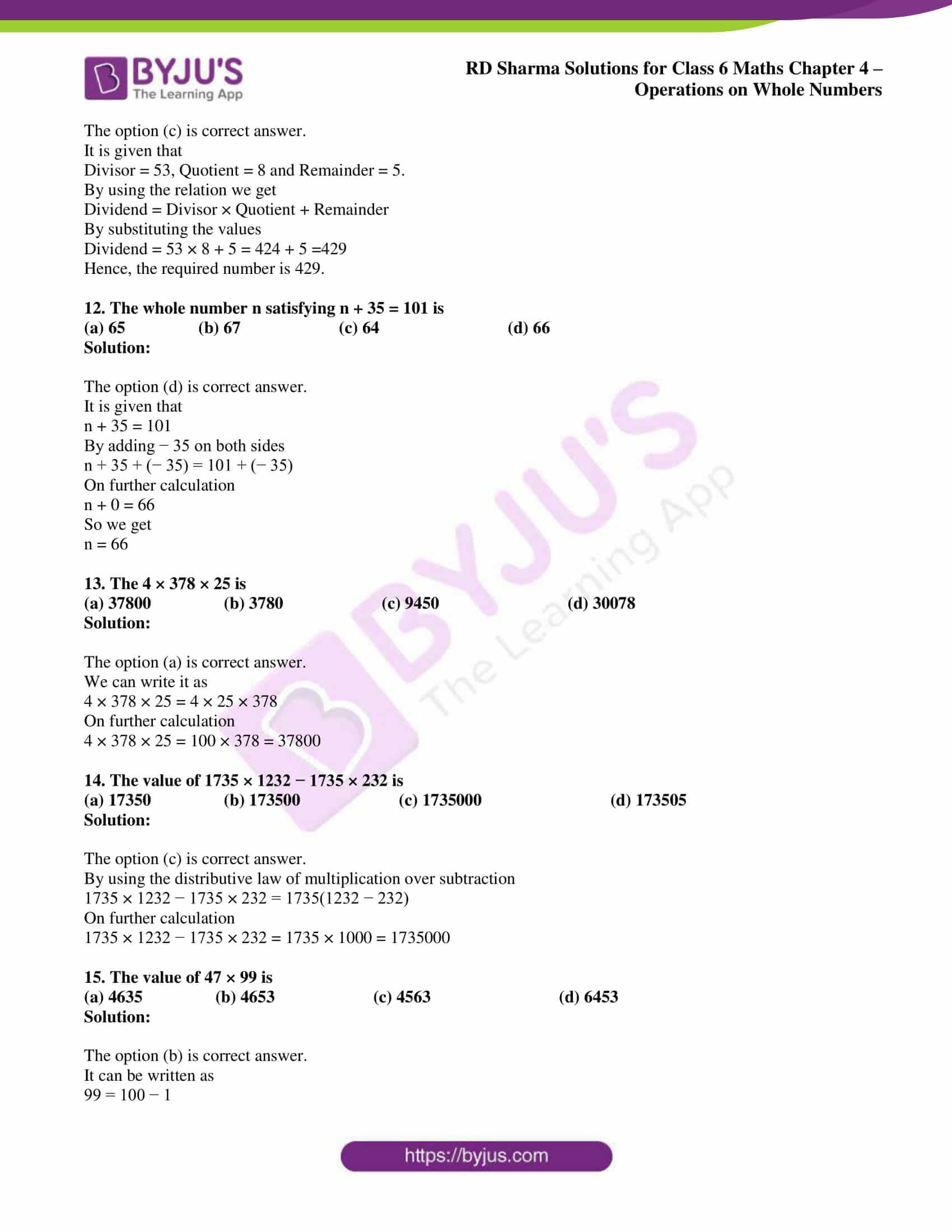# RD Sharma Solutions for Class 6 Maths Chapter 4: Operations on Whole Numbers Objective Type Questions

Objective Type Questions present at the end of Chapter 4 help students self analyse their knowledge about the concepts covered in the entire chapter. These questions are answered in RD Sharma Solutions for Class 6 by expert Mathematicians to help students perform well in the exam. The important formulas and shortcuts used in solving problems are highlighted, which help students grasp them faster. RD Sharma Solutions for Class 6 Maths Chapter 4 Operations on Whole Numbers Objective Type Questions PDF are available here.

## RD Sharma Solutions for Class 6 Maths Chapter 4: Operations on Whole Numbers Objective Type Questions Download PDF### Access answers to Maths RD Sharma Solutions for Class 6 Chapter 4: Operations on Whole Numbers Objective Type Questions

Mark the correct alternative in each of the following:

1. Which one of the following is the smallest whole number?
(a) 1                     (b) 2                         (c) 0                                     (d) None of these

Solution:

The option (c) is correct answer.

We know that the set of whole numbers is {0, 1, 2, 3, 4 …}.

Hence, the smallest whole number is 0.

2. Which one of the following is the smallest even whole number?
(a) 0                               (b) 1                                   (c) 2                                      (d) None of these

Solution:

The option (c) is correct answer.

We know that the natural numbers along with 0 form the collection of whole numbers.

Hence, the numbers 0, 1, 2, 3, 4 … form the collection of whole numbers.

So the number which is divisible by 2 is an even number and 2 is the smallest even number.

3. Which one of the following is the smallest odd whole number?
(a) 0                               (b) 1                                   (c) 3                                      (d) 5

Solution:

The option (b) is correct answer.

We know that the natural numbers along with 0 form the collection of whole numbers.

Hence, the numbers 0, 1, 2, 3, 4 … form the collection of whole numbers.

So the natural number which is not divisible by 2 is called an odd whole number and 1 is the smallest odd whole number.

4. How many whole numbers are between 437 and 487?
(a) 50                               (b) 49                                   (c) 51                                      (d) None of these

Solution:

The option (b) is correct answer.

We know that the whole numbers between 437 and 487 are 438, 439, 440, 441, …, 484, 485 and 486.

In order to find the required number of whole numbers subtract 437 from 487 and then subtract again 1.

Hence, there are (487 − 437) − 1 whole numbers lying between 437 and 487.

So we get (487 − 437) − 1 = 50 − 1 = 49

5. The product of the successor of 999 and the predecessor of 1001 is
(a) one lakh                            (b) one billion                               (c) one million                                (d) one crore

Solution:

The option (c) is correct answer.

We know that the successor of 999 = 999 + 1 = 1000

So the predecessor of 1001 = 1001 − 1 = 1000

It can be written as

Product of them = (Successor of 999) × (Predecessor of 1001)

By substituting the values

Product of them = 1000 × 1000 = 1000000 = one million

6. Which one of the following whole numbers does not have a predecessor?
(a) 1                            (b) 0                              (c) 2                               (d) None of these

Solution:

The option (b) is correct answer.

We know that the numbers 0, 1, 2, 3, 4 … form the collection of whole numbers.

Hence, the smallest whole number is 0 which does not have a predecessor.

7. The number of whole numbers between the smallest whole number and the greatest 2-digit number is
(a) 101                            (b) 100                              (c) 99                               (d) 98

Solution:

The option (d) is correct answer.

We know that the smallest whole number = 0

So the greatest 2 digit whole number = 99

Whole numbers which lie between 0 and 99 are 1, 2, 3, 4,…, 97, 98.

In order to find the number of whole numbers between 0 and 99, first subtract 1 from the difference of 0 and 99.

So the number of whole numbers between 0 and 99 = (99 − 0) − 1 = 99 − 1 = 98

8. If n is a whole number such that n + n = n, then n =?
(a) 1                            (b) 2                              (c) 3                               (d) None of these

Solution:

The option (d) is correct answer.

We know that 0 + 0 = 0, 1 + 1 = 2, 2 + 2 = 4….

Hence, the statement n + n = n is true only when n = 0.

9. The predecessor of the smallest 3-digit number is
(a) 999                            (b) 99                              (c) 100                               (d) 101

Solution:

The option (b) is correct answer.

We know that the smallest 3 digit number = 100

So the predecessor of 3 digit number = 100 − 1 = 99

10. The least number of 4-digits which is exactly divisible by 9 is
(a) 1008                            (b) 1009                              (c) 1026                               (d) 1018

Solution:

The option (a) is correct answer.

We know that the least 4-digit number = 1000

Hence, the least 4-digits which is exactly divisible by 9 is 1000 + (9 − 1) = 1008

11. The number which when divided by 53 gives 8 as quotient and 5 as remainder is
(a) 424                 (b) 419                       (c) 429                              (d) None of these

Solution:

The option (c) is correct answer.

It is given that

Divisor = 53, Quotient = 8 and Remainder = 5.

By using the relation we get

Dividend = Divisor × Quotient + Remainder

By substituting the values

Dividend = 53 × 8 + 5 = 424 + 5 =429

Hence, the required number is 429.

12. The whole number n satisfying n + 35 = 101 is
(a) 65                 (b) 67                       (c) 64                              (d) 66

Solution:

The option (d) is correct answer.

It is given that

n + 35 = 101

By adding − 35 on both sides

n + 35 + (− 35) = 101 + (− 35)

On further calculation

n + 0 = 66

So we get

n = 66

13. The 4 × 378 × 25 is
(a) 37800                 (b) 3780                       (c) 9450                              (d) 30078

Solution:

The option (a) is correct answer.

We can write it as

4 × 378 × 25 = 4 × 25 × 378

On further calculation

4 × 378 × 25 = 100 × 378 = 37800

14. The value of 1735 × 1232 − 1735 × 232 is
(a) 17350                 (b) 173500                       (c) 1735000                              (d) 173505

Solution:

The option (c) is correct answer.

By using the distributive law of multiplication over subtraction

1735 × 1232 − 1735 × 232 = 1735(1232 − 232)

On further calculation

1735 × 1232 − 1735 × 232 = 1735 × 1000 = 1735000

15. The value of 47 × 99 is
(a) 4635                 (b) 4653                       (c) 4563                              (d) 6453

Solution:

The option (b) is correct answer.

It can be written as

99 = 100 − 1

So we get

47 × 99 = 47 × (100 − 1)

On further calculation

47 × 99 = 47× 100 – 47 = 4700 – 47 = 4653

Hence, the value of 47 × 99 is 4653.